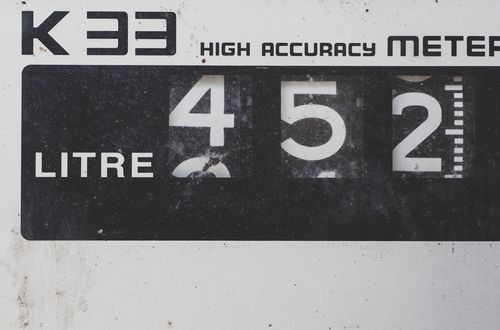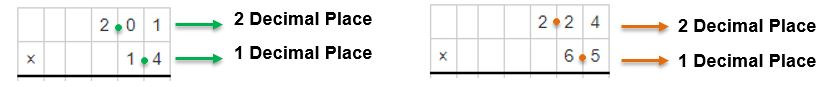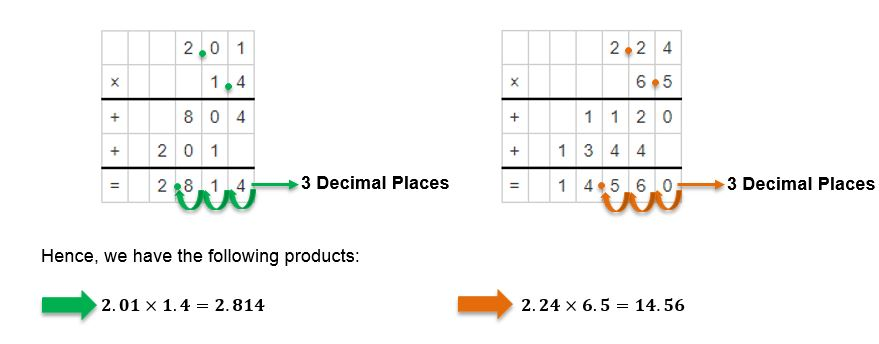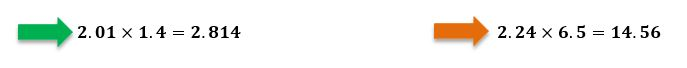# How to multiply decimals

Want to find out how to multiply decimals? We've provided a step-by-step guide of how to multiply decimals to teach you everything you need to know.## Step 1: Count the number of decimal places

Count the total number of decimal places found on the given factors. For example, if we want to find the product of 12.45 and 3.6, we first count the total number of decimal places.

## Step 2: Multiply the factors

Multiply the two factors as if the decimal points are not present. So, we find the product of 1245 and 36.

## Step 3: Move the total number of decimal places

Move the same number (from Step 1) of decimal places to the left of the product. For 12.45 and 3.6 , move three decimal places to the left of 44820.

## Examples of how to multiply decimals

Q1) Determine the product of the following factors. a. 2.01×1.4 b. 2.24×6.5

Count the total number of decimal places found in both factors.The total decimal places would be 2+1=3. This means that once we have the product, we move three decimal places to the left.Hence, we have the following products:#### Join today

The fastest way to practice

Unlock our complete testing platform and improve faster that ever.